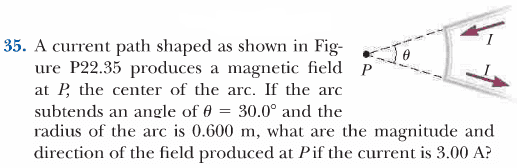# Magnetic field, current with arced path

iRaid

## Homework Statement## The Attempt at a Solution

$$B=\frac{\mu_{0}I}{4\pi}\int \frac{dl\times \hat{r}}{r^{2}}=\frac{\mu_{0}I}{4\pi r^{2}}\int dl\times \hat{r}$$
So I think since when you cross dl with r, you end up with just dl.
$$\frac{\mu_{0} I}{4\pi r^{2}}\int dl$$
l=rθ so dl=rdθ, substituting:
$$\frac{\mu_{0} I}{4\pi r^{2}}\int rd\theta=\frac{\mu_{0} I}{4\pi r}\int d\theta=\frac{\mu_{0} I}{4\pi r}\theta$$
Plugging in values I end up with: 2.618x10-7 T

Just need someone to look over work/logic, I think it's correct.

## Answers and Replies

iRaid
Just realized I could of just done the integral at ∫dl and plugged in rθ after, but whatever. Same result.

Homework Helper
Gold Member
Magnitude is correct.

Direction?